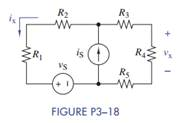Chat Now

# (a) Formulate mesh-current equations for the circuit in Figure P3−18. (b) Solve for vx and ix...

### (a) Formulate mesh-current equations for the circuit in Figure P3−18. (b) Solve for vx and ix...

(a) Formulate mesh-current equations for the circuit in Figure P3−18.

(b) Solve for vx and ix when R= 2:7 kΩ, R2 = 1.5 kΩ, R3 = 680 Ω, R= 2.2 kΩ, R5 = 3:3 kΩ, iS = 10 mA, and vS = 12 V.

(c) Use Multisim to verify your results in part (b) and then find the total power dissipated in the circuit.manish jayant 28-Jul-2020Get solution